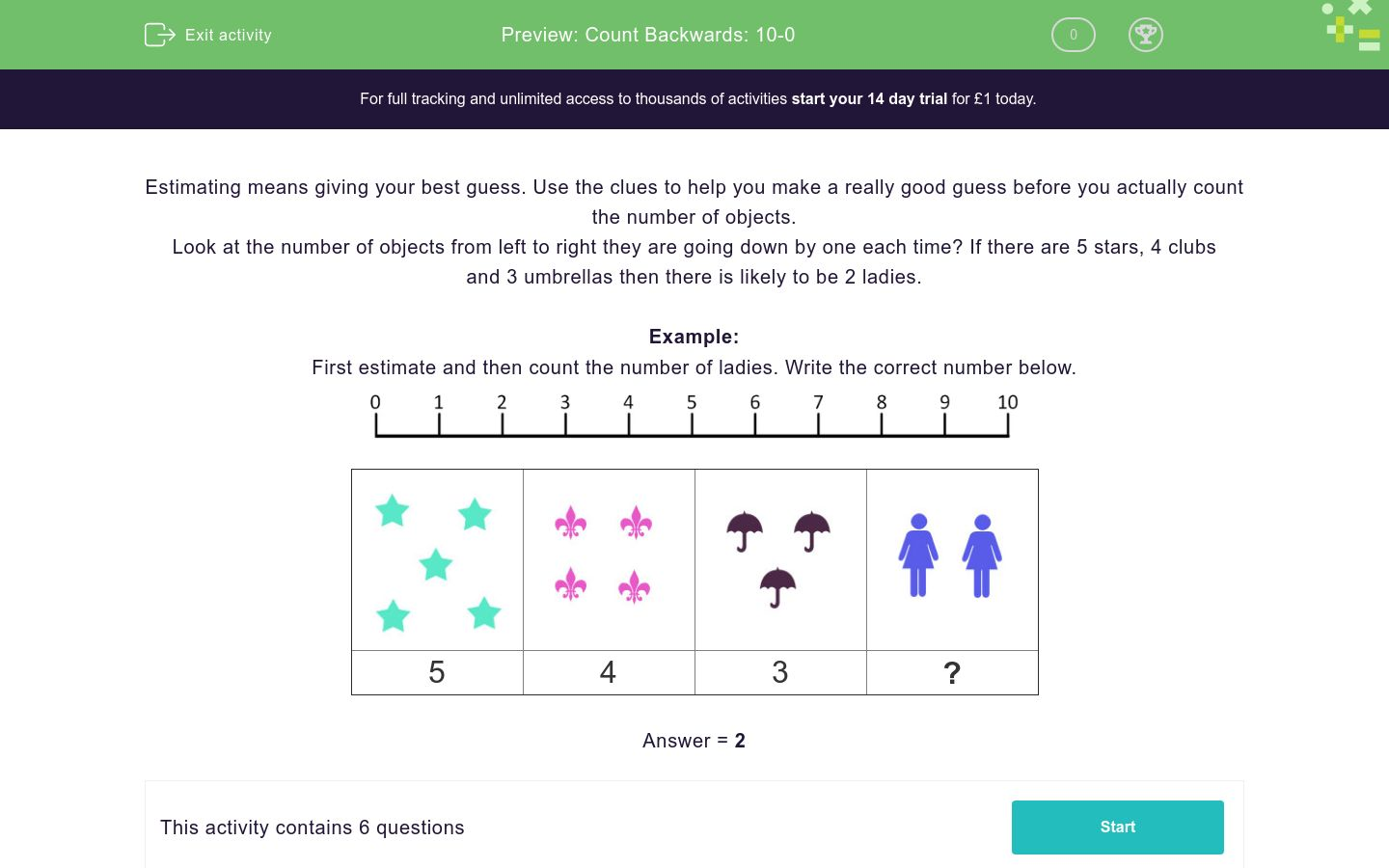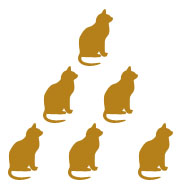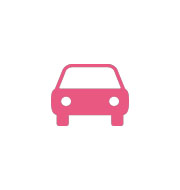# Count Backwards: 10-0

In this worksheet, students count backwards from 10 to 0, filling in any missing numbers in the sequence.Key stage:  KS 1

Curriculum topic:   Number: Number and Place Value

Curriculum subtopic:   Count to 100

Difficulty level:### QUESTION 1 of 10

Estimating means giving your best guess. Use the clues to help you make a really good guess before you actually count the number of objects.

Look at the number of objects from left to right they are going down by one each time? If there are 5 stars, 4 clubs and 3 umbrellas then there is likely to be 2 ladies.

Example:

First estimate and then count the number of ladies. Write the correct number below.5 4 3 ?

First estimate and then count the number of umbrellas. Write the correct number below.6 5 4 ?

First estimate and then count the number of clubs. Write the correct number below.6 5 ? 3

First estimate and then count the number of umbrella's. Write the correct number below.6 5 4 ?

First estimate and then count the number of stars. Write the correct number below.? 4 3 2

First estimate and then count the number of cars. Write the correct number below.4 3 2 ?

First estimate and then count the number of stars. Write the correct number below.6 ? 4 3
• Question 1

First estimate and then count the number of umbrellas. Write the correct number below.6 5 4 ?
3
EDDIE SAYS
There are 3 umbrella's.
• Question 2

First estimate and then count the number of clubs. Write the correct number below.6 5 ? 3
4
EDDIE SAYS
There are 4 clubs.
• Question 3

First estimate and then count the number of umbrella's. Write the correct number below.6 5 4 ?
3
EDDIE SAYS
There are 3 umbrellas.
• Question 4

First estimate and then count the number of stars. Write the correct number below.? 4 3 2
5
EDDIE SAYS
There are 5 stars.
• Question 5

First estimate and then count the number of cars. Write the correct number below.4 3 2 ?
1
EDDIE SAYS
There is 1 car.
• Question 6

First estimate and then count the number of stars. Write the correct number below.6 ? 4 3
5
EDDIE SAYS
There are 5 stars.
---- OR ----

Sign up for a £1 trial so you can track and measure your child's progress on this activity.

### What is EdPlace?

We're your National Curriculum aligned online education content provider helping each child succeed in English, maths and science from year 1 to GCSE. With an EdPlace account you’ll be able to track and measure progress, helping each child achieve their best. We build confidence and attainment by personalising each child’s learning at a level that suits them.

Get started